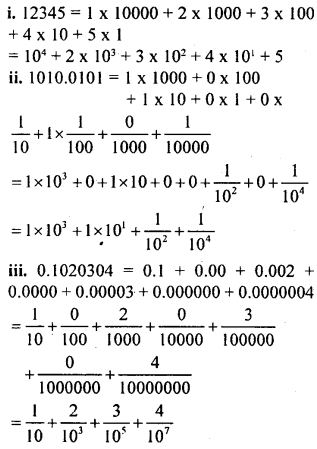# KSEEB Solutions for Class 8 Maths Chapter 10 Exponents Ex 10.1

Students can Download Maths Chapter 10 Exponents Ex 10.1 Questions and Answers, Notes Pdf, KSEEB Solutions for Class 8 Maths helps you to revise the complete Karnataka State Board Syllabus and score more marks in your examinations.

## Karnataka Board Class 8 Maths Chapter 10 Exponents Ex 10.1

Question 1.
Express the following numbers in the exponential form.

i. 1728
ii. $$\frac { 1 }{ 512 }$$
iii. 0.000169
i. 1728 = 12 x 12 x 12
1728 = 123

ii.$\frac{1}{512}=\frac{1}{2^{9}}=2^{-9}$

iii. 0.000169 = (0.013)-2

Question 2.
Write the following numbers using base 10 and exponents.
i. 12345
ii. 1010.0101
iii. 0.1020304